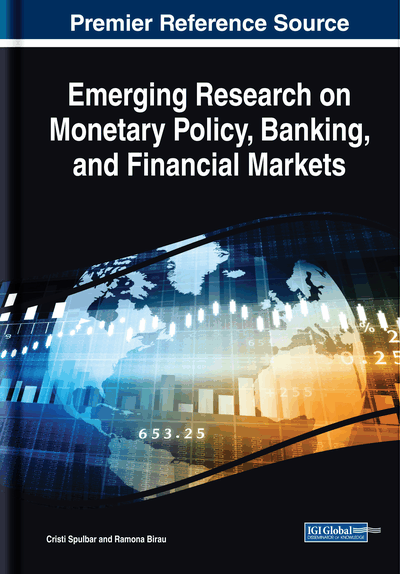# Estimating Long-Term Volatility on National Stock Exchange of India

DOI: 10.4018/978-1-5225-9269-3.ch011

## Abstract

The main objective of this chapter is to provide an elaborate framework on the long-term volatility of the National Stock Exchange of India based on Generalized Autoregressive Conditional Heteroskedasticity (GARCH) models. The CNX-100 index is one of the most diversified Indian stock indices which includes over 38 sectors of the economy. This stock index represents about 81.57% of the free-floating market capitalization of stocks listed on the National Stock Exchange (NSE) of India from March 2014. Moreover, this book chapter empirically tested volatility clusters of CNX100 index using a large sample database from October 2007 to July 2014.
Chapter Preview
Top

## Econometric Methodology

The main purpose of this subchapter is to investigate the estimation process of long-term volatility impact in National Stock Exchange of India using CNX 100 index. The econometric analysis includes applying Generalized Autoregressive Conditional Heteroskedistity which is also known as GARCH models (Bollerslev 1986). In this this subchapter, the econometric framework includes GARCH (1, 1) model, that is a combination of one ARCH term and one GARCH term. The GARCH (1, 1) model is capable to estimate volatility more particularly and measures the impact of shocks on financial market. GARCH (1, 1) model developed by Bollerslev and Taylor (1986) and which in detail mentioned below. The financial series stationarity is tested using Augmented Dickey Fuller test (ADF) and Kwiatkowski–Phillips–Schmidt–Shin test (KPSS). The empirical results are significant at level of 10%, 5% and 1% where 5% significant level considered for the further modeling process.

The financial time series consists of daily closing prices for sample stock index during the period between October 2007 to July 2014 with the exception of legal holidays or other events when stock markets haven’t performed transactions. In addition, the sample data series includes a total number of 1698 of daily observations. The continuously-compounded daily returns are calculated using the log-difference of the closing prices of stock market selected index, i.e. CNX 100 index as follows:

where p is the daily closing price.

The GARCH (1, 1) model was developed by Bollerslev and Taylor (1986). This model allows conditional variance of all variables to be dependent upon previous logs and it reflects on the entire financial time series. However, the first log of squared residual will be obtained from the mean equation and this presents idea about volatility from the previous time periods. The most widely used GARCH (1, 1) model is based on the following mathematical expression:

ht = ω + α1ut-12 + β1 ht-1

The empirical analysis is necessary to follow the hypothesis of stationary covariance as unconditional variance and to exist that it processed based on the following expressions:

The empirical analysis is necessary to follow the hypothesis of covariance stationary as unconditional variance and to exist that it processed based on the following expressions:

The research includes implementation of GARCH (1, 1) model conditional variance equation:

and thus, it can simply take the following form, i.e.:

.

## Complete Chapter List

Search this Book:
Reset